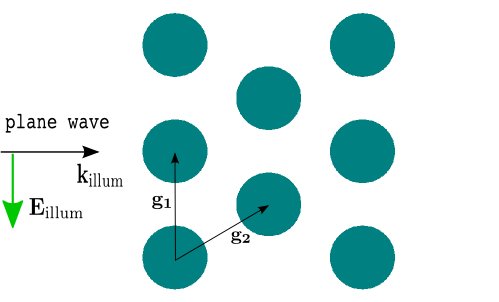# Loops¶

In this section we show how to exploit loops (for, while) within Matlab code snippets to define complicated geometries or project setups in a few number of script lines.

Loops within an embedded Matlab code block (cf. previous section Matlab Code Snippets) may extend over several blocks:

  1 2 3 4 5 6 7 8 9 10 11 12 13 14  Circle { Radius = 0.5 GlobalPosition = %(center)e ... } 

Here, a for-loop starts in line 2 within the first Matlab block. This loop is closed in line 13 after a .jcm code block (lines 6-10). Line 3 dynamically sets the value for key center, which serves as circle’s center in line 8. This way, we have defined ten circles, equally spaced in-direction.

To see how this works in practice, we want to compute the light scattering off multiple rods aligned on a hexagonal lattice. Figure “Multiple rod geometry” shows the setup.Multiple rod geometryandare called grid vectors. For a hexagonal alignment we havewith a lattice parameter, which is passed as a further parameter in the driver script:

  1 2 3 4 5 6 7 8 9 10 11 12 13 14 15 16 17 18 19 20 21 22 23 24 %% set problem parameter keys.radius = 0.3; keys.n_air = 1.0; keys.n_glass = 1.52; keys.lambda_0 = 0.550; % in um keys.polarization = 45; % in degree keys.a = 1.0; keys.n_rods = [3 3]; %% run the project results = jcmwave_solve('mie2D.jcmp', keys); %% get scattering cross section scattering_cross_section = ... results{2}.ElectromagneticFieldEnergyFlux{1}; fprintf('\nscattering cross section: %.8g\n', ... real(scattering_cross_section)); %% plot exported cartesian grid within matlab: cfb = results{3}; amplitude = cfb.field{1}; intensity = sum(conj(amplitude).*amplitude, 3); pcolor(cfb.X, cfb.Y, intensity); shading interp; view(0, 90); axis equal; 

In line 8 we set the number of rods in- and- direction. Figure “Intensity” shows the computed intensity of the electric field.Intensity

Pseudo-color intensity plot as produced by the driver run.m.

For this problem you again find a driver run_geo.m which only runs the mesh generation:

%% set geometry parameters
keys.radius = 0.3;
keys.a = 1.0;
keys.n_rods = [3 3];

%% generate mesh file only
jcmwave_geo('.', keys);

%% open grid.jcm in JCMview
jcmwave_view('grid.jcm');


You can use this script to “play” with the geometry parameters and to watch how the geometry is updated.

In the following we want to discuss the updated layout file:

  1 2 3 4 5 6 7 8 9 10 11 12 13 14 15 16 17 18 19 20 21 22 23 24 25 26 27 28 29 30 31 32 33 34 35 36 37 38 39 40 41 42 43 44 45 46 47 48 49 50 51 52 53 54 55 56 57 58  Layout { UnitOfLength = 1e-6 MeshOptions { MaximumSidelength = 0.1 } # Computational domain Parallelogram { DomainId = 1 Width = %(computational_domain_X)e Height = %(computational_domain_Y)e # set transparent boundary conditions BoundarySegment { BoundaryClass = Transparent } } # Scatterer (rod) Circle { DomainId = 2 Radius = %(radius)e GlobalPosition = %(center)e RefineAll = 4 } } 

The first Matlab block computes the grid vectors(lines 2-4), and adapts the computational domain size to enclose all rods (lines 6-11). There, maxX and maxY are the dimensions of the array of rods inand.

The Matlab block from lines 34-42 defines two for loops over the number of rods inand. Line 39 sets the center of the current rod, which is used in line 46 in the enclosed .jcm block (center_array is used to shift the center of the array of rods to the origin). The for loops are closed in the last Matlab block (lines 51-54).